﻿ 基于ARIMA模型的能源价格指数分析与预测 Analysis and Forecast of the Energy Price Index Based on ARIMA Model

Statistics and Application
Vol.07 No.02(2018), Article ID:24590,7 pages
10.12677/SA.2018.72022

Analysis and Forecast of the Energy Price Index Based on ARIMA Model

Yanwen Yan

College of Science, China University of Petroleum, Beijing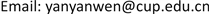Received: Apr. 2nd, 2018; accepted: Apr. 20th, 2018; published: Apr. 27th, 2018ABSTRACT

In this paper, the monthly international market energy price index of 2000-2017 year is used as the raw data. Through the series of data processing, the ARIMA(1,1,0) model is established. And the international market energy price index time series of the changes in the performance of the law is expounded; the international market energy price index for the next 12 months is forecasted. The test results show that the model can make a short-term reasonable prediction for the future, and can give decision-making reference for economic development goals.

Keywords:ARIMA Model, Index, Non-Stationary, PredictionCopyright © 2018 by author and Hans Publishers Inc.1. 引言

ARIMA模型全称为求和自回归移动平均模型(Autoregressive Integrated Moving Average Model)，简记ARIMA，是由博克思(Box)和詹金斯(Jenkins)提出的著名时间序列预测方法，称为Box-Jenkins模型。其中ARIMA(p,d,q)称为求和自回归移动平均模型，AR是自回归，p是自回归项数，MA为移动平均，q为移动平均项数，d为时间序列成为平稳是所做的差分次数。所谓ARIMA模型，是指将非平稳时间序列转化为平稳时间序列，然后将因变量仅对它的滞后值以及随机误差项的现值和滞后值进行回归所建立的模型  。

2. 理论基础

2.1. 平稳性检验

2.2. 纯随机性检验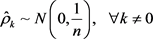${Q}_{LB}=n\left(n+2\right)\sum _{k=1}^{m}\left(\frac{{\stackrel{^}{\rho }}_{k}^{2}}{n-k}\right)~{\chi }^{2}\left(m\right)$

${\text{Q}}_{LB}$ 统计量的真值大于程 ${x}_{1-\alpha }^{2}$ 分位点，或该统计量的P值小于 $\alpha$ 时，则以 $1-\alpha$ 的置信水平拒绝原假设，认为该序列为非纯随机序列；否则，接受原假设，认为该序列为纯随机序列。

2.3. ARIMA模型

$\left\{\begin{array}{c}\Phi \left(B\right){\nabla }^{d}{x}_{t}=\Theta \left(B\right){\epsilon }_{t}\\ E\left({x}_{t}\right)=0,Var\left({\epsilon }_{t}\right)={\sigma }_{\epsilon }^{2},E\left({\epsilon }_{t}{\epsilon }_{s}\right)=0,s\ne t\\ E\left({x}_{t}{\epsilon }_{s}\right)=0,\forall s

ARIMA(p,d,q)模型中参数d是非平稳时间序列经过差分的次数。从理论上讲，足够多次的差分运算可以充分地提取序列中的非平稳确定性信息，但差分运算的阶数并不是越多越好。因为差分运算是一种对信息的提取、加工过程， 每次差分都会有信息的损失，所以在实际应用中差分运算的阶数要适当，应当避免过差分的现象。

ARIMA(p,d,q)模型的实质是差分运算与ARMA模型的结合，具有较高的短期预测精度，且不需要数据具有较强的结构性，只需要从数据本身寻找规律，并能较好的拟合数据。

3. 实例分析

ARIMA模型建模遵循如下流程图：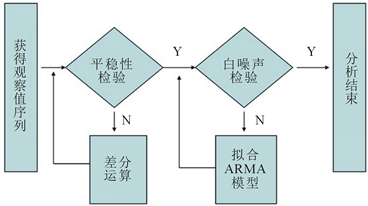1) 运用国际市场能源价格指数数据，基于SAS软件绘制其时序图  ：

2) 对原序列进行差分运算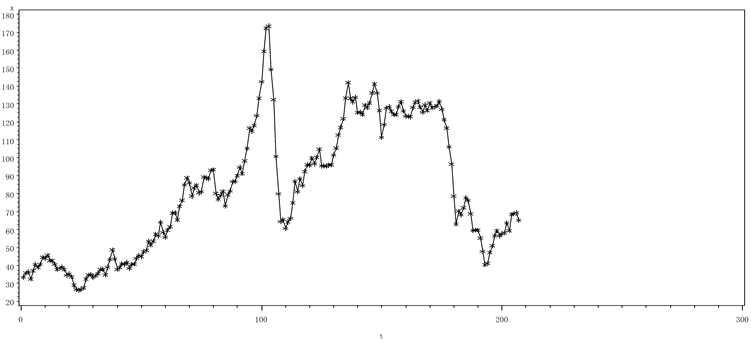Figure 1. The sequence diagram of the international market energy price index from January 2000 to March 2017 year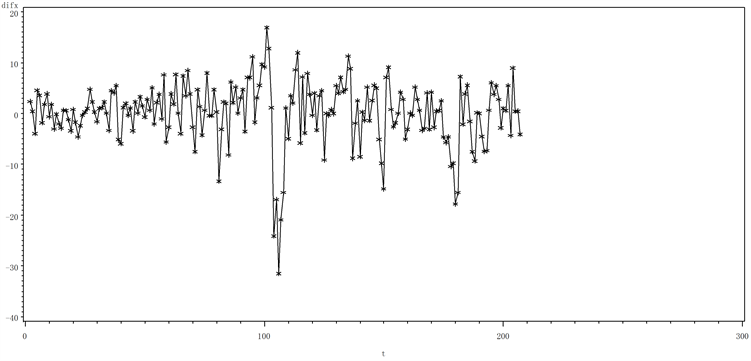Figure 2. The sequence diagram of the first order difference of the energy price index from January 2000 to March 2017 year

3) 对平稳的1阶差分序列进行白噪声检验Figure 3. The diagram of autocorrelation of the first order difference of the energy price index seriesTable 1. The test of white noise of the first order difference of the energy price index seriesTable 2. The parameter estimation of the first order difference of the energy price index series

4) 对非平稳非白噪声差分序列拟合ARMA模型

5) 预测结果Table 3. The prediction value of the fitting model ARIMA(1,1,0)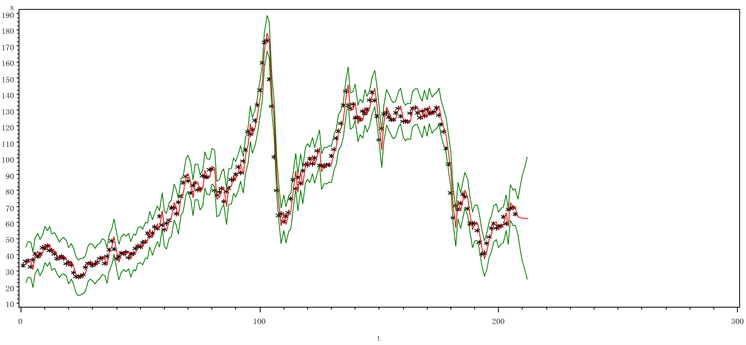说明：图中星号为序列观察值；中间曲线为序列预测值；上下曲线为95%的置信区间。

Figure 4. The prediction diagram of the energy price index series

4. 总结

Analysis and Forecast of the Energy Price Index Based on ARIMA Model[J]. 统计学与应用, 2018, 07(02): 176-182. https://doi.org/10.12677/SA.2018.72022

1. 1. 王燕. 时间序列分析[M]. 第四版. 北京: 中国人民大学出版社, 2016.

2. 2. 唐炼. 世界能源供需现状与发展趋势[J]. 国际石油经济, 2005(1): 30-33.

3. 3. 区诗德, 覃思乾. 基于60分钟线的ARIMA模型分析日K线走势[J]. 统计与决策, 2005, 12(24): 145-146.

4. 4. 高惠璇, 等. SAS系统SAS/ETS软件使用手册[M]. 北京: 中国统计出版社, 1998.